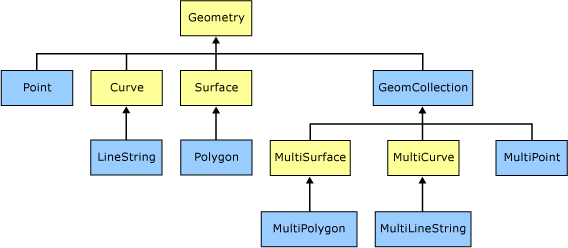# Types of Spatial Data

There are two types of spatial data. The geometry data type supports planar, or Euclidean (flat-earth), data. The geometry data type conforms to the Open Geospatial Consortium (OGC) Simple Features for SQL Specification version 1.1.0.

In addition, SQL Server supports the geography data type, which stores ellipsoidal (round-earth) data, such as GPS latitude and longitude coordinates.

The geometry and geography Data Types support eleven spatial data objects, or instance types. However, only seven of these instance types are instantiable; you can create and work with these instances (or instantiate them) in a database. These instances derive certain properties from their parent data types that distinguish them as Points, LineStrings, Polygons, or as multiple geometry or geography instances in a GeometryCollection.

The figure below depicts the geometry hierarchy upon which the geometry and geography data types are based. The instantiable types of geometry and geography are indicated in blue.As the figure indicates, the seven instantiable types of the geometry and geography data types are Point, MultiPoint, LineString, MultiLineString, Polygon, MultiPolygon, and GeometryCollection. The geometry and geography types can recognize a specific instance as long as it is a well-formed instance, even if the instance is not defined explicitly. For example, if you define a Point instance explicitly using the STPointFromText() method, geometry and geography recognize the instance as a Point, as long as the method input is well-formed. If you define the same instance using the STGeomFromText() method, both the geometry and geography data types recognize the instance as a Point.

For more information about these instances, see the following topics:

## Differences Between the Two Data Types

The two types of spatial data often behave quite similarly, but there are some key differences in how the data is stored and manipulated.

### How Connecting Edges Are Defined

The defining data for LineString and Polygon types are vertices only. The connecting edge between two vertices in a geometry type is a straight line. However, the connecting edge between two vertices in a geography type is a short great elliptic arc between the two vertices. A great ellipse is the intersection of the ellipsoid with a plane through its center and a great elliptic arc is an arc segment on the great ellipse.

### Measurements in Spatial Data Types

In the planar, or flat-earth, system, measurements of distances and areas are given in the same unit of measurement as coordinates. Using the geometry data type, the distance between (2, 2) and (5, 6) is 5 units, regardless of the units used.

In the ellipsoidal, or round-earth system, coordinates are given in degrees of latitude and longitude. However, lengths and areas are usually measured in meters and square meters, though the measurement may depend on the spatial reference identifier (SRID) of the geography instance. The most common unit of measurement for the geography data type is meters.

### Orientation of Spatial Data

In the planar system, the ring orientation of a polygon is not an important factor. For example, a polygon described by ((0, 0), (10, 0), (0, 20), (0, 0)) is the same as a polygon described by ((0, 0), (0, 20), (10, 0), (0, 0)). The OGC Simple Features for SQL Specification does not dictate a ring ordering, and SQL Server does not enforce ring ordering.

In an ellipsoidal system, a polygon has no meaning, or is ambiguous, without an orientation. For example, does a ring around the equator describe the northern or southern hemisphere? If we use the geography data type to store the spatial instance, we must specify the orientation of the ring and accurately describe the location of the instance.

SQL Server 2008 places the following restrictions on using the geography data type:

• Each geography instance must fit inside a single hemisphere. No spatial objects larger than a hemisphere can be stored.

• Any geography instance from an Open Geospatial Consortium (OGC) Well-Known Text (WKT) or Well-Known Binary (WKB) representation that produces an object larger than a hemisphere throws an ArgumentException.

• The geography data type methods that require the input of two geography instances, such as STIntersection(), STUnion(), STDifference(), and STSymDifference(), will return null if the results from the methods do not fit inside a single hemisphere. STBuffer() will also return null if the output exceeds a single hemisphere.

### Outer and Inner Rings Not Important in geography Data Type

The OGC Simple Features for SQL Specification discusses outer rings and inner rings, but this distinction makes little sense for the SQL Server geography data type: any ring of a polygon can be taken to be the outer ring.

For more information on OGC specifications, see the following:

OGC Specifications, Simple Feature Access Part 1 - Common Architecture

OGC Specifications, Simple Feature Access Part 2 – SQL Options